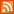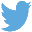Bucaro TecHelpHTTPS Encryption not required because no account numbers orpersonal information is ever requested or accepted by this siteCustom Search# Binary Subtraction with Example JavaScript Code

When working in the familiar base 10 number system you can re-imagine the mathematical operation 12 - 5 as 12 + (-5). This is useful when plotting an operation on the number line, because then you need only one set of rules (for addition). On the number line you would start at +12 and because the 5 is negative you would move 5 units to the left, ending up at the +7 mark.

Similarly, with binary mathematics you can re-imagine the operation 1100 - 101 as 1100 + 011 (the 2s complement of 101). If we perform the binary operation 1010 - 10001, the result is a negative number and we would indicate this by setting a sign bit to 1. Of course you could subtract a binary number using the borrow method, but this would require more complicated hardware and software.

The sign bit is set when the processor detects that the subtrahend is larger than the minuend. Don't confuse the sign bit with the MSB (Most Significant Bit) carry bit, which you get only with a positive result.

The first step in a binary subtraction is to replace the subtrahend with its 2's complement. This is done by replacing each 1 with 0 and replacing each 0 with 1, and then adding 1. But many people do this wrong because they don't understand the importance of magnitude. Before you complement the subtrahend, make the minuend and the subtrahend the same length.

Shown below is example code to make the the minuend and the subtrahend the same length.

```var magnitude = 0;
if(minuend.length > subtrahend.length)
{
magnitude = minuend.length;
var magdif = magnitude - subtrahend.length;
for(var k = 0; k < magdif; k++)
{
}
}
else
{
magnitude = subtrahend.length;
var magdif = magnitude - minuend.length;
for(var k = 0; k < magdif; k++)
{
}
}
```

The code creates a string strPad containing the required number of 0s to pad the shorter value out to the same length as the longer value, concatenates the shorter value on to the padding and then replaces the original value with the padded value. Now your ready to complement the subtrahend.

Shown below is example code to complement the subtrahend.

```var strComp = "";
for(var i = 0; i < subtrahend.length; i++)
{
if(subtrahend.charAt(i) == 0)
{
strComp += 1;
}
else
{
strComp += 0;
}
}
```

The code creates an empty string and then loops through the characters of the subtrahend, concatenating a 1 on the string when it finds an 0 in the subtrahend and concatenating an 0 when it finds a 1. This creates the complement, now you need to add 1 to it to create the 2's complement.

 .menubtn { margin-top:10px; margin-left:50px; width:220px; opacity:1; border-style:solid; border-color:#009000; background-color:#66ff66; transition-property: opacity; transition-duration: 1s; transition-timing-function: ease-out; } .menubtn:hover { opacity:1; background-color:#e5ff23; } Menu - More Java Script ProgrammingRSS FeedFollow @Stephen Bucaro[Site User Agreement] [Privacy Policy] [Site map] [Search This Site] [Contact Form]
Copyright©2001-2021 Bucaro TecHelp 13771 N Fountain Hills Blvd Suite 114-248 Fountain Hills, AZ 85268